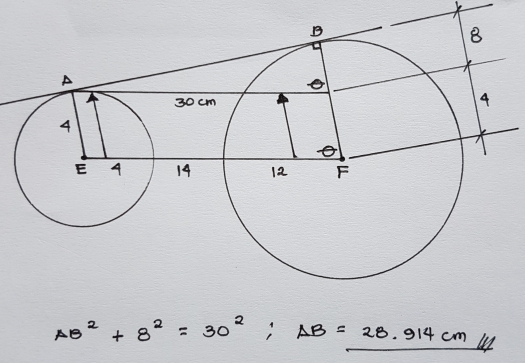# Circles

5 posts / 0 new
MONGOL○482
Circles

Two circles have radius of 4cm. and 12cm. respectively. If the distance between their centers is 30cm., compute the length of the common external tangent to the two circles on one side only.
It says the answer is 31.05cm.

The following image is my solution but as you can see i got 28.914cm. Please help me. I cant seem to find where did i make a mistake. Thanks a ton sir!Jhun VertYour solution is correct. But there are two possible external tangents that can be drawn. I don’t know if it can also be called as external tangent, but I guess it is.

Jhun VertTry the red one.Sorry for my drawing, I am in my phone only.

MONGOL○482

Thank you sir for the verification!!

However,the inner tangent line (red line) i believe is called internal tangent. I tried solving it though (30^2=x^2 +16^2) just maybe i might get the 31.05cm answer that the book wants but got a different answer.

Jhun VertYes you are right, the red line is internal tangent. The answer key is not correct, it is for

$\sqrt{30^2 + 8^2} = 31.05 ~ \text{cm}$